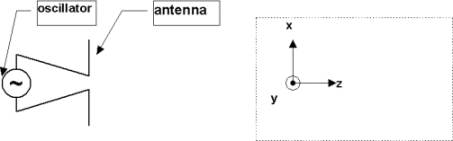Teaching Physics with the Physics Suite Edward F. Redish

Tracking a plane wave in a box

An oscillating current is producing an electromagnetic wave. The region shown enclosed by a dotted box (not to scale) is far from the current. In it, the field produced is well approximated by a plane wave traveling in the z direction and having its E-field pointed along the x-direction (using the coordinate system shown).1. I perform a series of measurements of the electric field at the origin and obtain a result which points in the x-direction and is well represented by the function

E(t) = E0 cos(wt).

What result would I find if instead of at the origin, I repeated my experiment at the point with coordinates {0,0,z}? Explain how you know.

2. What result would I get if I made my measurements at the point in the box with coordinates {2, 3, z}cm. (The point is still well within the dotted box.)

3. For what values of z would I find exactly the same result as I found at the origin?

Note to the instructor: The standard picture of an EM plane wave (with sinusoidal graphs attached perpendicular to the axis of travel in order to represent electric and magnetic fields) is deeply confusing to many students. They assume either that the field is only present along the axis or that it exist only for the spatial extent of the sinusoid. This last is a very surprising approach to a physicist, since the graphs plotted do not have dimensions of lengths. Students in the introductory course usually ignore dimensions and suffer the consequences of serious confusion.

Not finding what you wanted? Check the Site Map for more information.